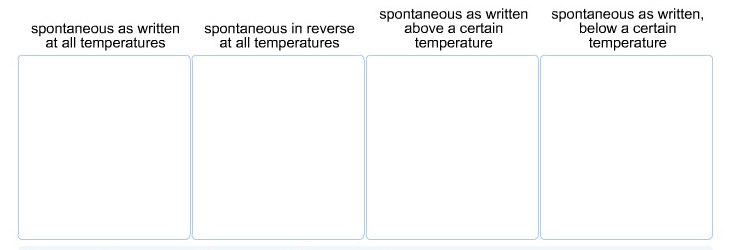# Problem: Classify the possible combinations of signs for a reaction's Δ H and Δ S values by the resulting spontaneity. ΔH = -                     ΔH = +                      ΔH = +                ΔH = -                           ΔS = -                     Δ S = +                      Δ S = -                Δ S = +

###### FREE Expert Solution

Recall that we can determine the spontaneity of the reaction based on the signs of ΔH and ΔS

We can use the Punnett square shown below:###### Problem Details

Classify the possible combinations of signs for a reaction's Δ H and Δ S values by the resulting spontaneity.ΔH = -                     ΔH = +                      ΔH = +                ΔH = -

ΔS = -                     Δ S = +                      Δ S = -                Δ S = +